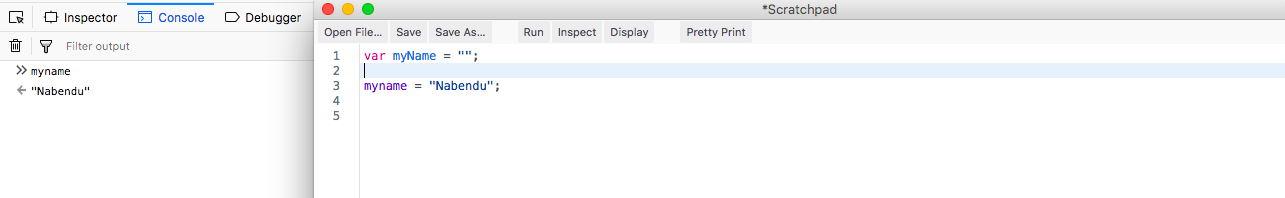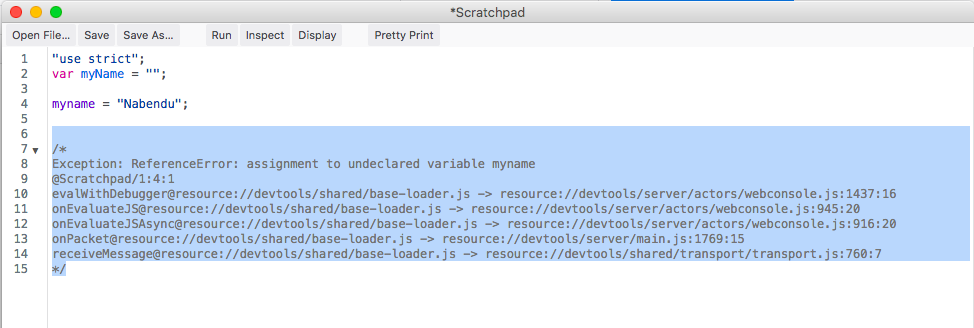Welcome to part-15 of the series and the first part in Tricky questions.

Now, the interviewer can ask some really tricky questions mainly related to JavaScript. There answers are not generally what they seems.

Question 96- What is wrong in using `typeof bar === “object”` to determine if bar is an object? Answer- There is nothing wrong in using `typeof bar === “object”` to determine if “bar” is an object.

But there is an old bug in JavaScript, which is that null is also considered as Object.

``````var bar = null;
console.log(typeof bar === "object"); //true
``````

So, we should avoid this in our logic by adding addtional checks for null.

``````var bar = null;
console.log((bar !== null) && (typeof bar === "object")); //false
``````

Question 97- What is the output of the below code and why?

``````(function(){
var a = b = 3;
})();

console.log("b is " + b);
console.log("a is " + a);
``````

Answer-Since, both “a” and “b” are defined inside an IIFE, they shouldn’t be available outside.

But in fact var a = b = 3;is shorthand for

``````var a = b;
b = 3;
``````

So, it means that “b” becomes global and it will result in 3, but “a” will give reference error. The output will be as below.

``````b is 3
Exception: ReferenceError: a is not defined
``````

Question 98- What is the output of the below code and why?

``````var myObject = {
foo: "bar",
func: function() {
var self = this;
console.log("outer func:  this.foo = " + this.foo);
console.log("outer func:  self.foo = " + self.foo);
(function() {
console.log("inner func:  this.foo = " + this.foo);
console.log("inner func:  self.foo = " + self.foo);
}());
}
};
myObject.func();
``````

Answer-The above code will output the below in console.

``````outer func:  this.foo = bar
outer func:  self.foo = bar
inner func:  this.foo = undefined
inner func:  self.foo = bar
``````

In the outer function, both “this” and “self” refers to “bar”. So, it will print “bar” in console. In the inner IIFE function, “this” refers to that function, so “this.foo” is undefined. But we can access “self.foo” as it’s scope is accesible because of var self = this decalared before inner IIFEE.

Question 99- What is the significance of wrapping the entire content of a JavaScript source file in a function block?Answer-This is a common practice employed by many popular JavaScript libraries like jQuery and Node.js. This technique creates a closure around the entire contents of the file which, perhaps most importantly, creates a private namespace and thereby helps avoid potential name clashes between different JavaScript modules and libraries.

Question 100- What is the main benefit of using ‘use strict’ at the beginning of JavaScript source file?Answer-Some of the javascript crazy behaviour like we can assign value to undeclared variable are not desired by many developers. We can restrict the same using strict mode introduced in Ecma Script 5.

Consider the below example, in which we declare a variable myName. And somewhere down our program we spell it wrong as myname and assign a value. So, what JS does is create a new variable myname and assign Nabendu o it. Clearly, not what we desire.Javascript crazy behaviour

So, we use the strict mode by writing “use strict” at the top of the program. And now if we run the program, it will give a run time error.use strict

Question 101- Consider the two below functions. Will they both return the same thing?

``````function foo1()
{
return {
bar: "hello"
};
}

function foo2()
{
return
{
bar: "hello"
};
}

console.log("foo1 returns:");
console.log(foo1());
console.log("foo2 returns:");
console.log(foo2());
``````

Answer-The output of the above code will be as below.

``````foo1 returns:
Object { bar: "hello" }
foo2 returns:
undefined
``````

Both of them should return the same thing ie Object { bar: “hello” }, but there is a bug in foo2. The reason is that semicolon are optional in JavaScript, so when the line containing return statement is encountered in foo2 a semicolon is inserted immediately after the return statement. And the return contains noting, so it return undefined.

Question 102- What is the output of the below code and why?

``````console.log(0.1 + 0.2);
``````

Answer-Numbers in JavaScript are all treated with floating point precision, and as such, may not always yield the expected results.

The above example demostrate this issue. It will print out :

``````0.30000000000000004
``````

Question 103- What is the output of the below code and why?

``````(function() {
console.log(1);
setTimeout(function(){console.log(2)}, 1000);
setTimeout(function(){console.log(3)}, 0);
console.log(4);
})();
``````

Answer-The output of the above code will be:

``````1
4
3
2
``````

It is because the setTimeout() is an asynchronous call and been handled by Browser API and Message Queue. So, even the setTimeout() with 0 ms waiting have to go through the whole Browser API and Message Queue, before been pushed to Call Stack to be shown. Also, the Call Stack needs to be completely empty before the SetTimeout() will be processed.

Details of the above Event Loop can be found in Question 5 of the link here.

Question 104- What a multiply method which works with both these below syntax?

``````console.log(multiply(2,3));   // Outputs 6
console.log(multiply(2)(3));  // Outputs 6
``````

Answer-The second case can be solved with currying function and we can differ it with first case by using arguments.

``````function multiply(x) {
if (arguments.length == 2) {
return arguments * arguments;
} else {
return function(y) { return x * y; };
}
}
``````

Question 105- What is the output of the below code and why?

``````var arr1 = "john".split('');
var arr2 = arr1.reverse();
var arr3 = "jones".split('');
arr2.push(arr3);
console.log("array 1: " + arr1.slice(-1));
console.log("array 2: " + arr2.slice(-1));
``````

``````array 1: j,o,n,e,s
array 2: j,o,n,e,s
``````

Actually, arr1 and arr2 are same thing after we execute the above code ie `[‘n’,’h’,’o’,’j’, [‘j’,’o’,’n’,’e’,’s’] ]`

This happens because of the mutating effect of reverse(). When we do this line var arr2 = arr1.reverse();, it not only reverses arr1 but also arr2. Also, the reverse() makes arr2 a reference to arr1.

Now, in this line arr2.push(arr3);we are passing an entire array to push. So, our arr2 will become `[‘n’,’h’,’o’,’j’, [‘j’,’o’,’n’,’e’,’s’] ]` and so do arr1.

Next, we are using slice with -1 to get the last element which is a complete array i.e. j,o,n,e,s

Question 106- What is the output of the below code and why?

``````console.log(1 +  "2" + "2");
console.log(1 +  +"2" + "2");
console.log(1 +  -"1" + "2");
console.log(+"1" +  "1" + "2");
console.log( "A" - "B" + "2");
console.log( "A" - "B" + 2);
``````

``````122
32
02
112
NaN2
NaN
``````

The issue is that JavaScript is a loosely typed language and it performs automatic type conversion on values to accommodate the operation being performed. The explanation is below:

Eg 1: On moving left to right the first operation is 1 + “2” , since one is string , so JavaScript perform a string conversation on 1 and the equation becomes “1” + “2” which equals to “12” on string concatination. Next the operation “12” + “2” will also result in string concatenation and produce “122”. Eg 2: The first operation performed will be the unary operation of +”2". The unary operation converts it to numeric 2 and then the operation 1 + 2 yields 3. But the next operation 3 + “2” will yield 32 because of string convertion of 3 and then concatenation. Eg 3: The explanation is simlar to above(Eg 2) and the unary operation changes -”1" to -1. So, 1–1 will result in 0. Then 0 + “2” will result in “02” Eg 4: Although the first +”1" is converted into numeric 1, due to unary operator. But then 1 + “1” will result in “11” due to string conversion and concatenation. Finally “11” + “2” gets displayed as “112” Eg 5: Since minus(-) operator cannot be applied to string and “A” and “B” cannot be converted to numeric value, “A” — “B” yields NaN(Not a Number). Now, NaN + “2” will yield “NaN2” because of string concatenation. Eg 6: The output of “A”-”B” is NaN as explained above(Eg 5). But any numeric value applied to NaN also yields NaN. So, NaN + 2 will give NaN.

Question 107- What is the output of the below code and why?

``````for (var i = 0; i < 5; i++) {
setTimeout(function() { console.log(i); }, i * 1000 );
}
``````

Answer-The output logged will be: 5 5 5 5 5 and not 0 1 2 3 4

The explaination is that since var is not block scoped and each function executed within the loop will be executed after the entire loop has completed. Due to var the value of “i” will become 5 for all five calls.

Question 108- What is the output of the below code and why?

``````console.log("0 || 1 = "+(0 || 1));
console.log("1 || 2 = "+(1 || 2));
console.log("0 && 1 = "+(0 && 1));
console.log("1 && 2 = "+(1 && 2));
``````

``````0 || 1 = 1
1 || 2 = 1
0 && 1 = 0
1 && 2 = 2
``````

The explaination of the same is below : The or(||) Operator: In an expression of the form x || y, if x is evaluated to true then true(1) is returned. If x is evaluated to false then only y will be evaluated. Eg 1: In the expression 0 || 1, since 0 is false, we will check 1 which is true. So, 1 will be the output. Eg 2: In the expression 1 || 2, since 1 is true we won’t check the other part and will output 1.

The and(&&) Operator: In an expression of the form x && y, if x is evaluated to false then false(0) is returned. If x is evaluated to true then only y will be evaluated. However, one interesting thing of && operator is that when an expression is evaluated as “true”, then the expression itself is returned. Eg 3: In the expression 0 && 1, since 0 is false. So, 0 is returned and displayed and we are not checking 1. Eg 4: In the expression 1 && 2, both 1 is true and 2 is true. So, as per the special feature of && operator 2 will be returned and displayed.

This concludes the part-15 of the series and first part of Tricky questions.

This post is also available on DEV.